# 基于 webGL 的元素周期表 3D 交互展示

2019/04/10 10:10

## 界面展示

2D界面：整体是一个 ht.ui.SplitLayout 分割组件（左右分割），左边使用 ht.ui.HTView 包装了 GraphView 拓扑图组件，右边是一个 ht.ui.Form 表单组件。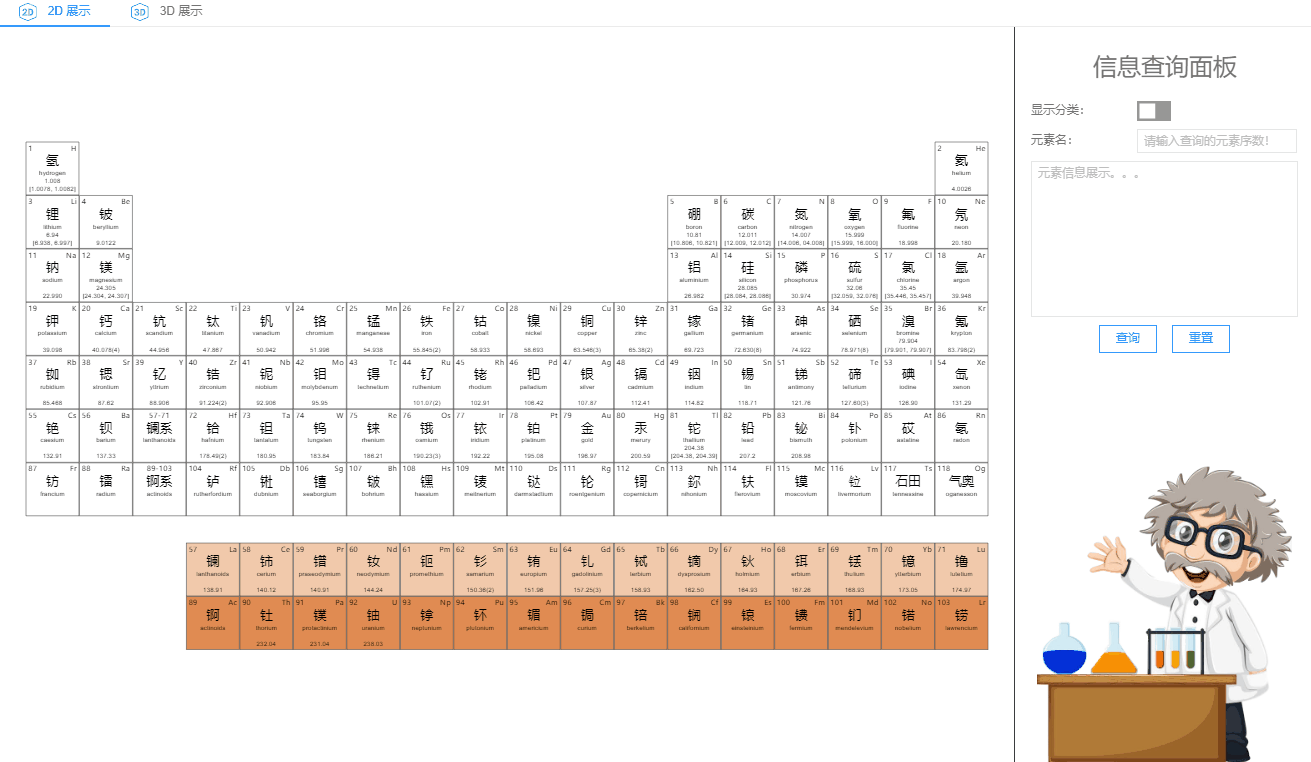3D界面：整体是一个 ht.ui.SplitLayout 分割组件（上下分割），上边添加了 ht.ui.HBoxLayout 构成的按钮组，下边是使用 ht.ui.HTView 包装了 Graph3dView 场景。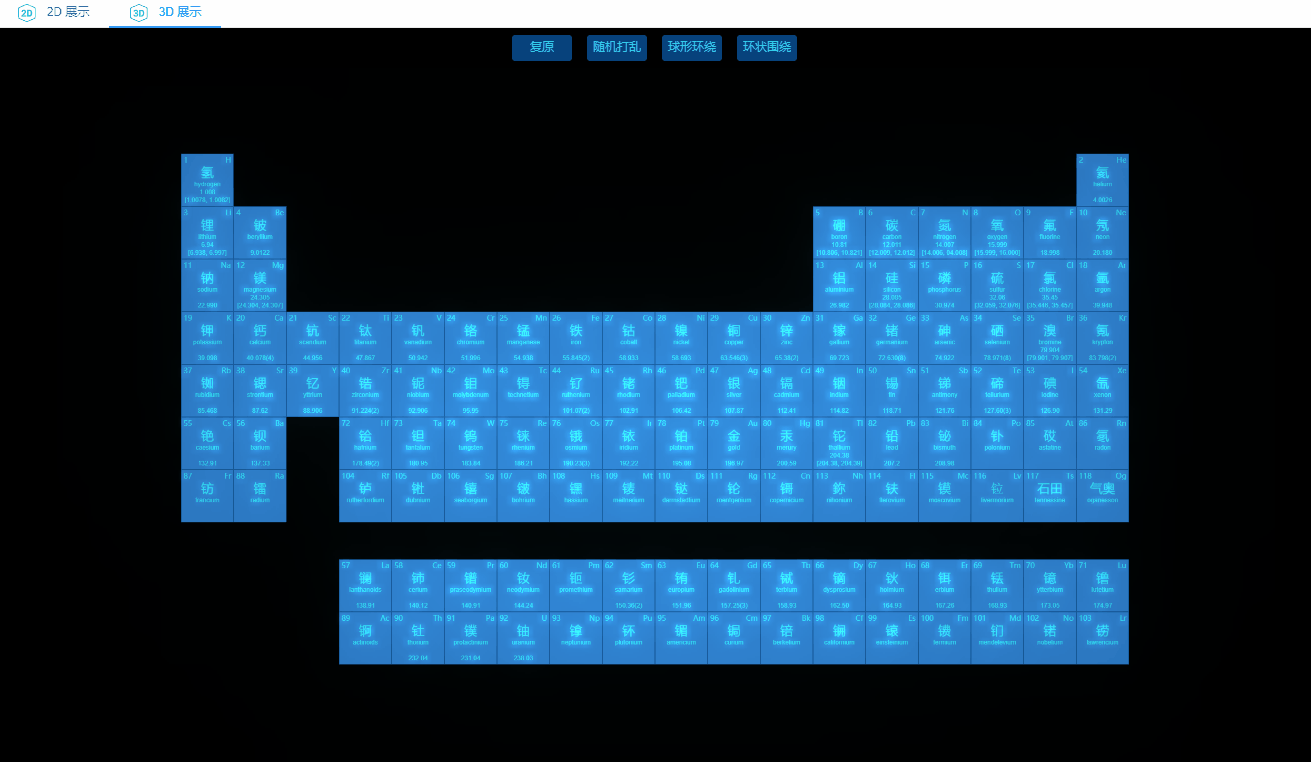## 2D 界面代码分析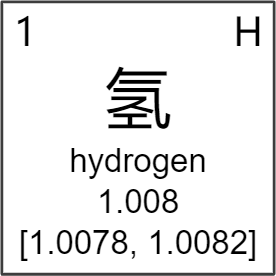1 toggle.on('p:selected', e => {
2     if (e.newValue) {
3         this.htView.legend.s('2d.visible', true); // 显示类别图例
4         this.htView.addClassification(); // 展示分类
5     }
6     else {
7         this.htView.legend.s('2d.visible', false); // 隐藏类别图例
8         this.htView.initElements(); // 原始样式
9     }
10 });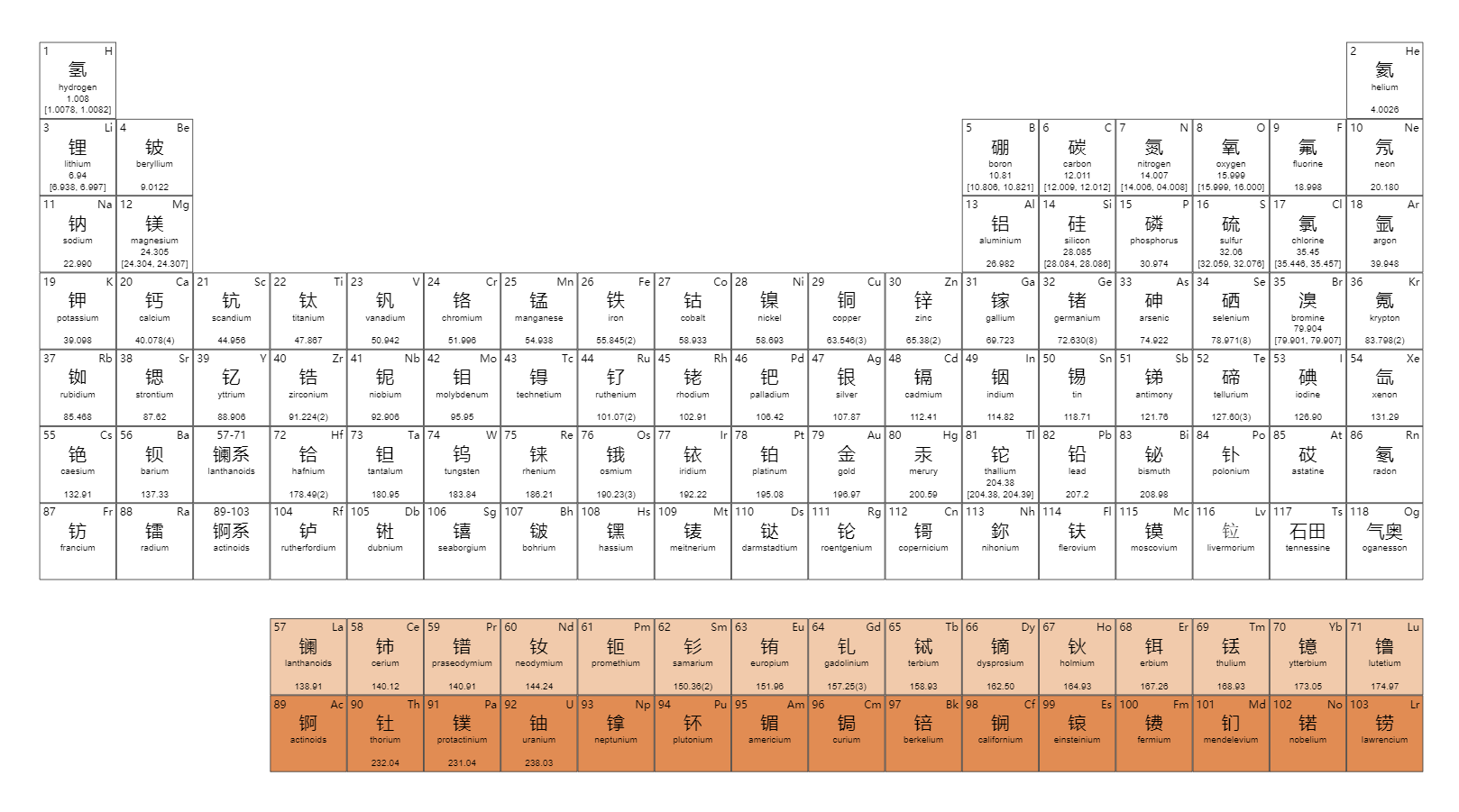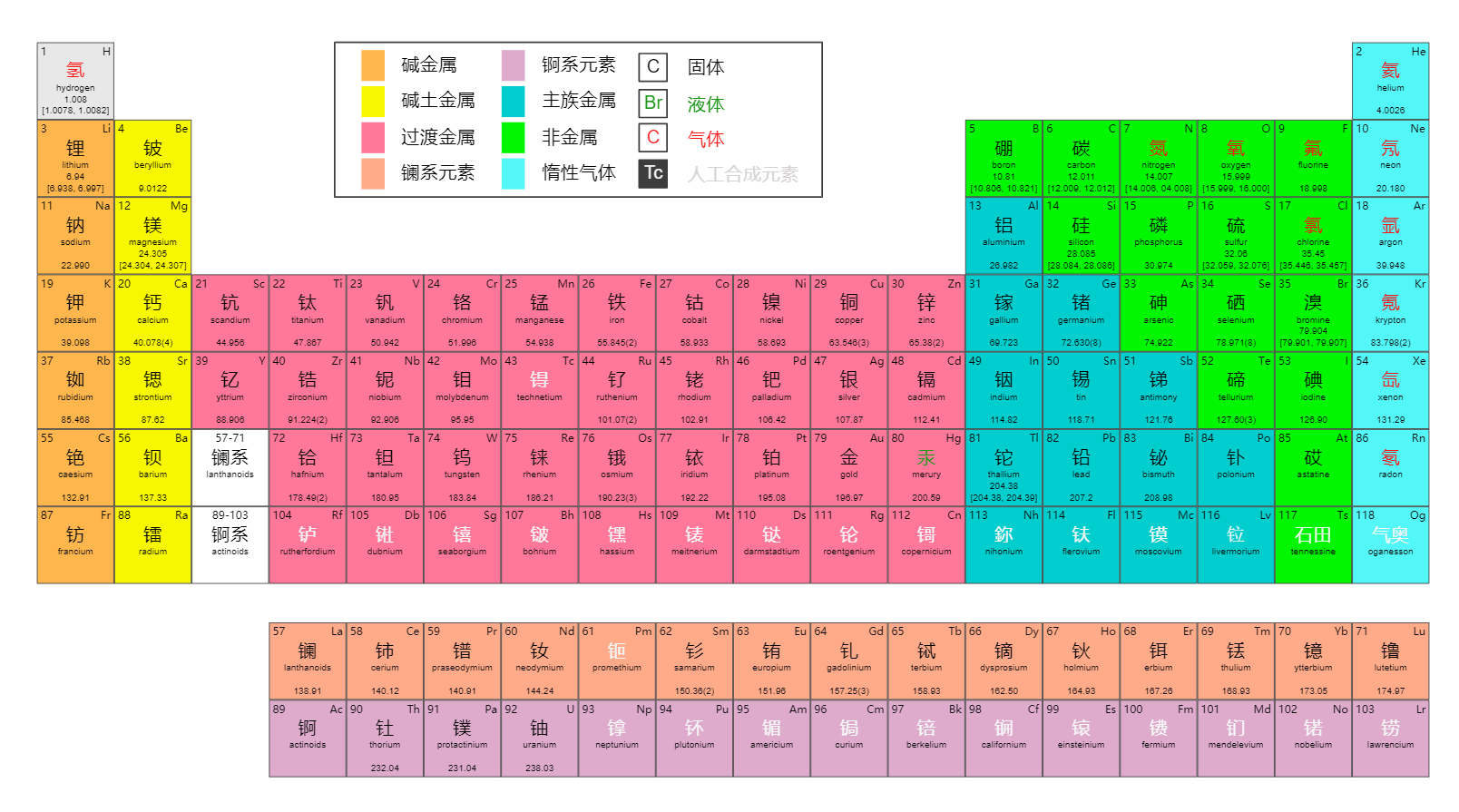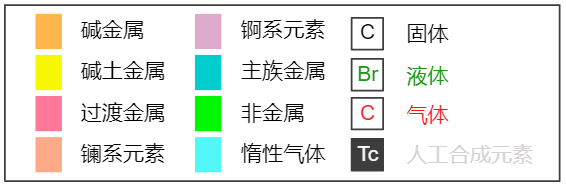1 let textField = new ht.ui.TextField();
2 textField.setFormDataName('textField'); // 设置在表单中的名称
3 textField.setPlaceholder('请输入查询的元素序数！');
4 textField.setMaskRe(/\d/); // 限制只能输入数字
5 textField.setInstant(true); // 开启即时模式，值改变就派发属性改变事件
6 textField.on('p:value', (e) => { // 监听值改变事件
7     let value = e.newValue;
8     if (value > 118) {
9         textField.setErrorMessage('只有 1 ~ 118 号元素哟！', {
10             placements: ['top']
11         });
12     }
13     else { textField.setErrorMessage(null); }
14 });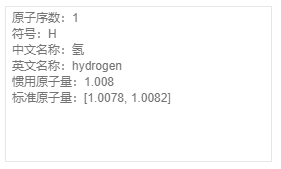## 3D 界面代码分析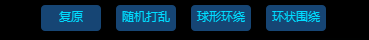1 createButton(text) {
2     let button = new ht.ui.Button();
3     button.setBorder(null);
4     button.setHoverBorder(null);
5     button.setActiveBorder(null);
6     button.setBackground(new ht.ui.drawable.ColorDrawable('rgba(37,115,194,0.6)', 4)); // normal 背景
7     button.setHoverBackground(new ht.ui.drawable.ColorDrawable('rgba(10,92,173,0.50)', 4)); // hover 背景
8     button.setActiveBackground(new ht.ui.drawable.ColorDrawable('rgba(15,132,250,0.6)', 4)); // active 背景
9     button.setText(text);
10     button.setTextColor('rgb(0, 211, 255)');
11     button.setHoverTextColor('rgb(0, 211, 255)');
12     button.setActiveTextColor('rgb(0, 211, 255)');
13
14     return button;
15 }

3D 场景

1 node.s({
2     'shape3d': 'billboard', // 设置节点类型为‘billboard’公告板
3     'shape3d.image': 'symbols/元素2.json', // 设置面片贴图
4     'shape3d.reverse.flip': true, // 设置反面是否显示正面内容
5     'shape3d.image.cache': true, // 进行贴图缓存
6     'shape3d.fixSizeOnScreen': false, // 设置是否固定保持屏幕大小，不随缩放而变化
7     'select.brightness': 1 // 设置选中亮度为 1
8 });

1. 随机打乱：设置一组空间范围值，生成范围内的（x,y,z）随机值，用以设置节点位置。

1 let dm = this.dm,
2     datasMap = {};
3
4 dm.each(data => {
5     let x = Math.random() * 2000 - 1000; // 获取随机 x
6     let y = Math.random() * 2000 - 1000; // 获取随机 y
7     let z = Math.random() * 500 - 250; // 获取随机 z
8
9     let position = data.getPosition3d(),
10         px = position,
11         py = position,
12         pz = position;
13
14     datasMap[data] = {
15         x: x,
16         y: y,
17         z: z,
18         px: px,
19         py: py,
20         pz: pz
21     };
22 });2. 球形环绕：绕球面螺旋线生成点坐标。

1 let dm = this.dm,
2     datas = dm.getDatas(),
3     datasMap = {};
4
5 let r = 400,
6     theta, phi;
7
8 for (let i = 0; i < 118; i++) {
9     let data = datas.get(i);
10     theta = (i + 1) / 118 * 180; // 获取球系坐标
11     phi = (i + 1) / 118 * 360 * 10; // 获取球系坐标
12 　　 // 球系坐标转换为 HT 三维坐标
13     let z = r * Math.sin(theta * Math.PI / 180) * Math.cos(phi * Math.PI / 180),
14         x = r * Math.sin(theta * Math.PI / 180) * Math.sin(phi * Math.PI / 180),
15         y = r * Math.cos(theta * Math.PI / 180);
16
17     let position = data.getPosition3d(),
18     px = position,
19     py = position,
20     pz = position;
21
22     datasMap[data] = {
23         x: x,
24         y: y,
25         z: z,
26         px: px,
27         py: py,
28         pz: pz
29     };
30 }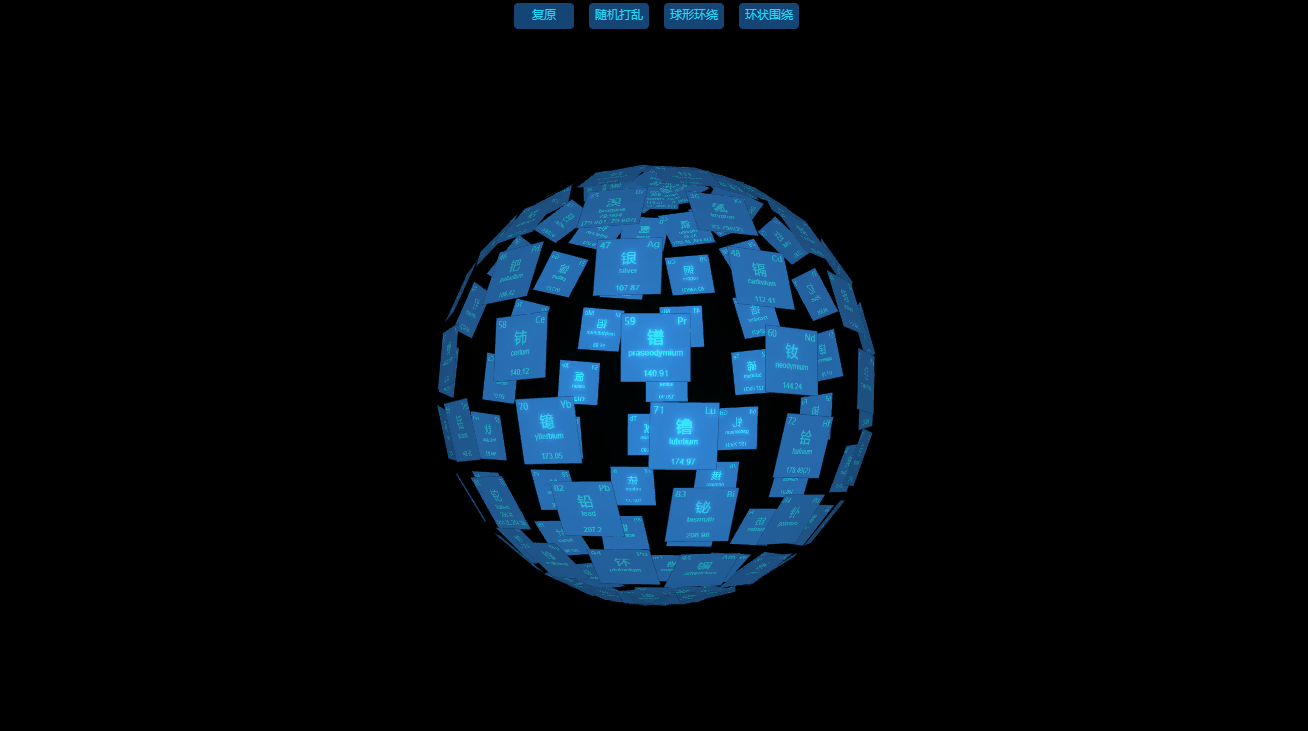3. 环形围绕：设置一个环绕半径、起始高度，以固定角度旋转，每次降低节点的设置高度。

1 let dm = this.dm,
2     datasMap = {},
3     datas = dm.getDatas(),
4     radius = 400,
5     angle = 18,
6     num = 360 / angle;
7
8 let y = 300,
9     count = 0;
10 for (let i = 0; i < 6; i++) {
11     for (let j = 0; j < num; j++) {
12         let data = datas.get(count),
13             radian = Math.PI / 180 * j * angle;
14
15         if (!data) break;
16         count++;
17
18         let x = radius * Math.cos(radian),
20
21         let position = data.p3(),
22             px = position,
23             py = position,
24             pz = position;
25
26         datasMap[data] = {
27             x: x,
28             y: y,
29             z: z,
30             px: px,
31             py: py,
32             pz: pz
33         };
34         y -= 6;
35     }
36 }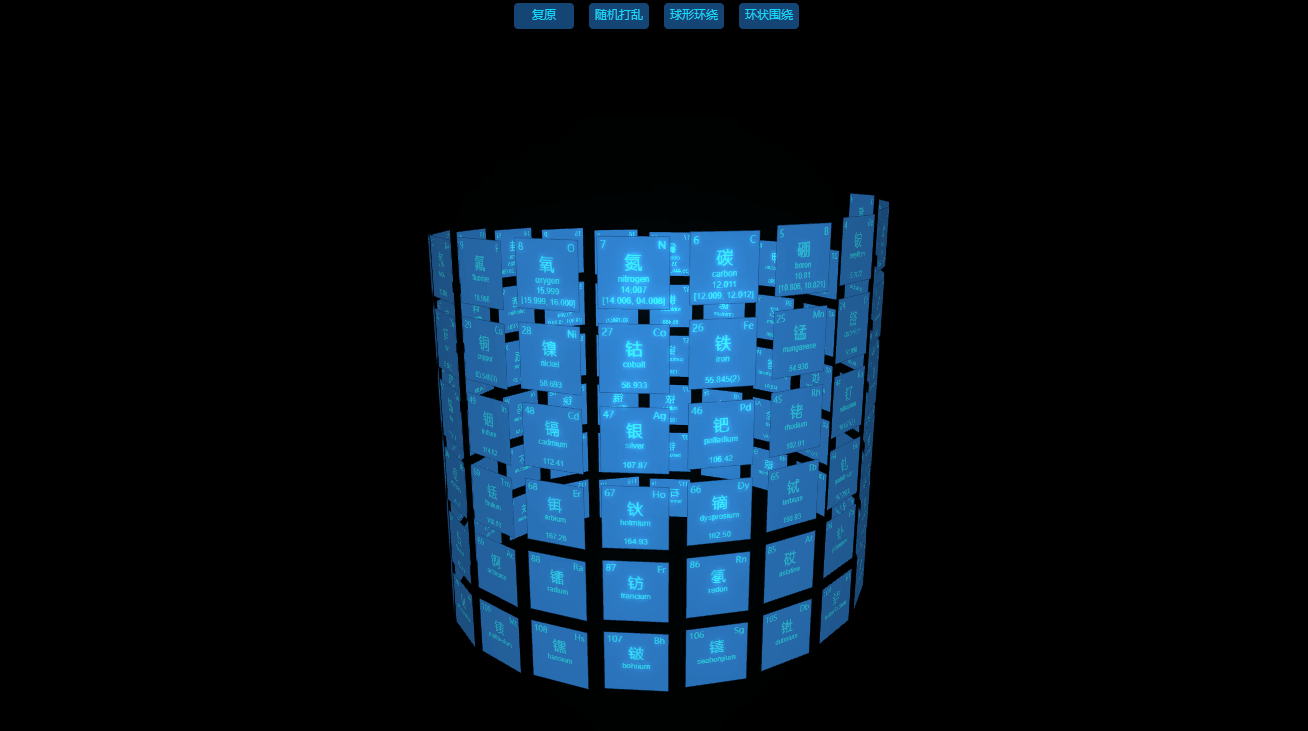4. 复原：根据记录的元素的行数和列数，计算元素节点的 xy 值，z 值固定。

1 let dm = this.dm,
2     datasMap = {};
3
4 dm.each(data => {
5     let index = data.a('index'),
6         row = data.a('row'),
7         col = data.a('col');
8
9     let position = data.getPosition3d(),
10         px = position,
11         py = position,
12         pz = position;
13
14     datasMap[data] = {
15         index: index,
16         row: row,
17         col: col,
18         px: px,
19         py: py,
20         pz: pz
21     };
22 });

##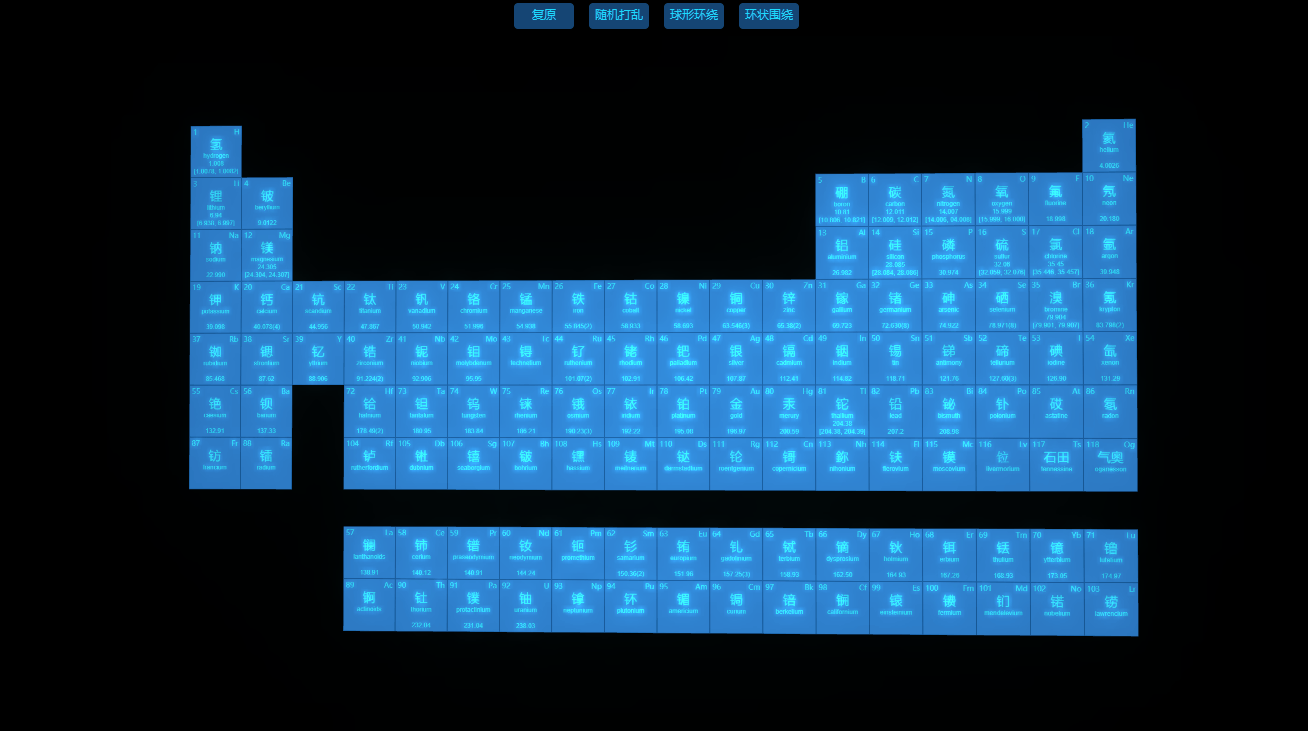5. 元素切换状态时的动画：详情了解入门手册动画

1 ht.Default.startAnim({
2     duration: 1500,
3     easing: function(t) {
4         return t * t;
5     },
6     action: function(v, t) {
7         dm.each(data => {
8             let info = datasMap[data],
9                 x = info.x,
10                 y = info.y,
11                 z = info.z,
12                 px = info.px,
13                 py = info.py,
14                 pz = info.pz;
15
16             data.p3(px + v * (x - px), py + v * (y - py), pz + v * (z - pz)); // 移动元素位置
17             data.lookAt([0, y, 0], 'back'); // 调整元素朝向
18         });
19     }
20 });

## 总结### 作者的其它热门文章

0
0 收藏

0 评论
0 收藏
0